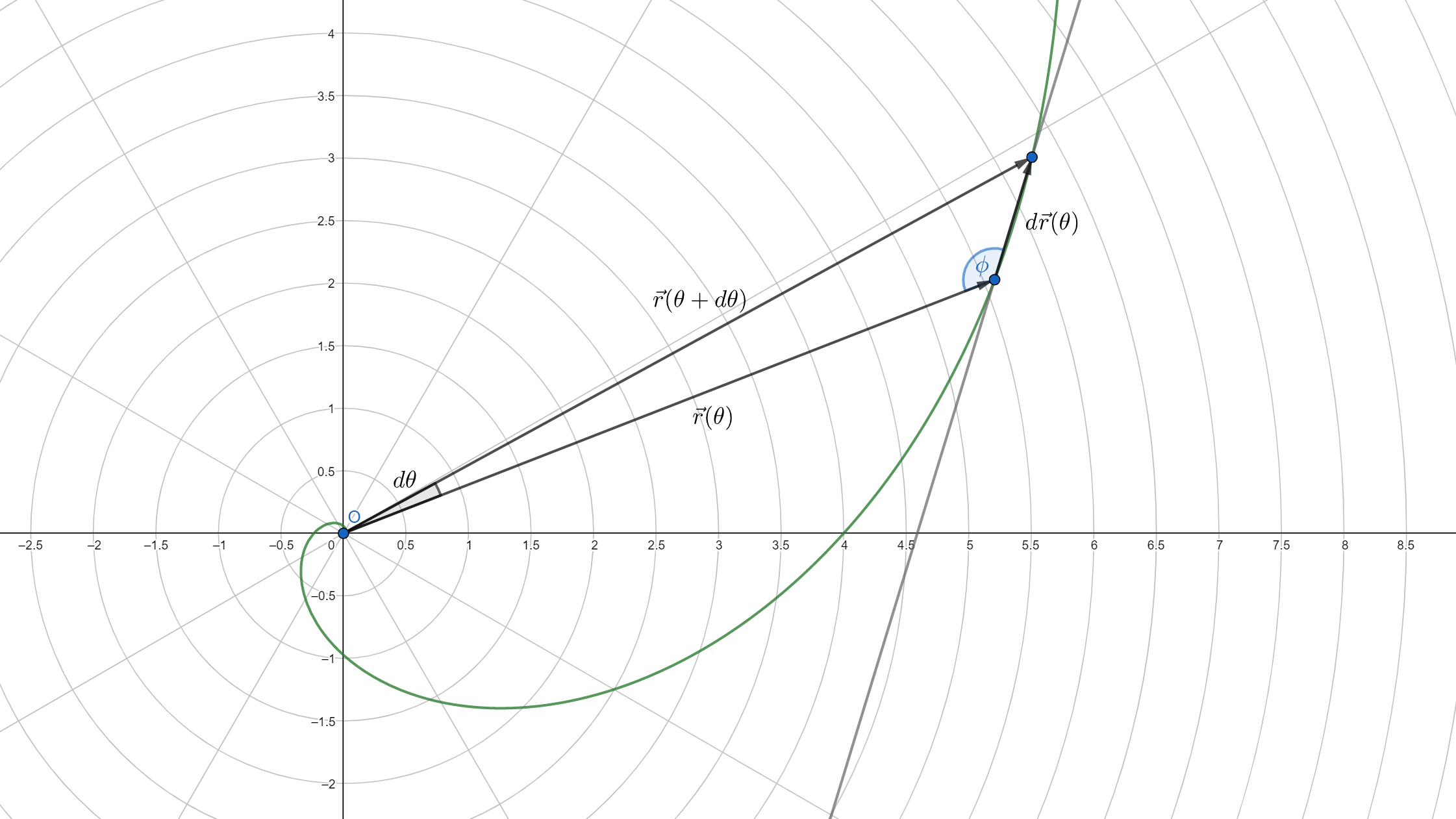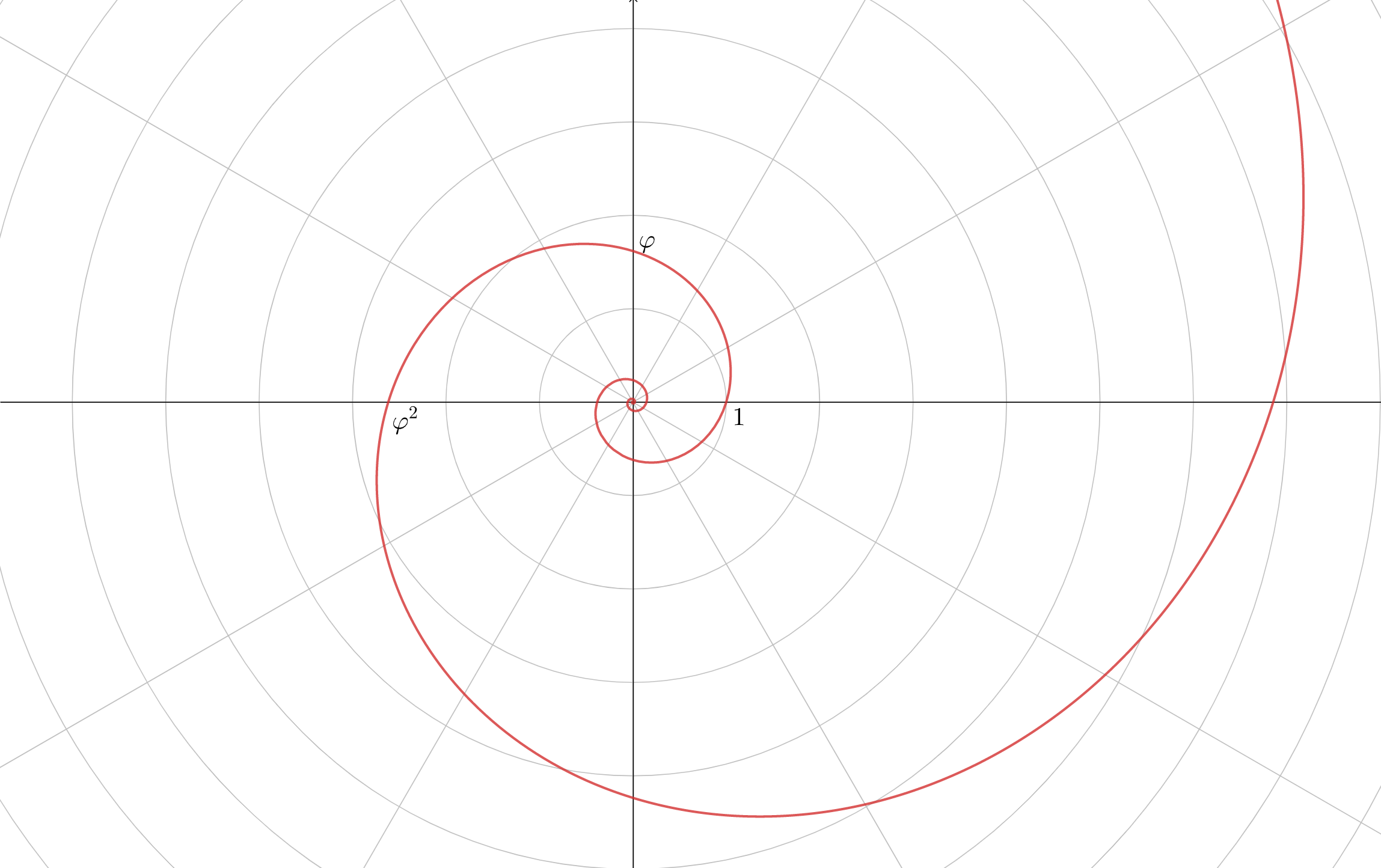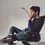# Spira mirabilis (Logarithmic spiral)

Hi folks,

Today, in daily challenges section, I saw that the subject of one discussion and related problem was Logarithmic spiral, a famous curve which often appear in nature and mathematical properties of which fascinated Jacob Bernouli so much that he named it Spira mirabilis - Latin for "miraculous spiral". I was, myself, for the first time introduced to this curve when I was reading Hamilton's Lectures on quaternions not while ago.Nautilus shell follows spira mirabilis. Photo credit: www.somanautiko.com

My wish is to supplement what was said and please a curious reader who was maybe left wondering how does the formula of Logarithmic spiral follow from properties used to describe it. The derivation below requires a little knowledge of analytic geometry and differential calculus.

We start off by defining the curve: radial line and tangent line at each point form an angle $\phi$ of a constant magnitude. There are a few ways to convert this statement into mathematical equation, here's one of them:

$\dfrac{\vec{r}(\theta) \cdot \vec{\dot{r}}(\theta)}{\left | \vec{r}(\theta) \right |\left| \vec{\dot{r}}(\theta) \right |} = \cos{\phi} = \text{const.}$

This equation says that we want dot product of radius vector and derivative of radius vector with respect to polar angle divided by their magnitudes to be a constant value, namely a cosine of the angle they form. Here, term derivative of radius vector with respect to polar angle corresponds to the ratio of displacement vector and infinitesimal change in polar angle. In order to make the notation somewhat cleaner, from now on, I will write $r$ instead of $\left| \vec{r}(\theta) \right |$ to denote the magnitude of the vector $\vec{r}(\theta)$. Consequently, $\dot{r}$ would denote derivative of the magnitude of the radius vector with respect to $\theta$.As $d\theta$ gets arbitrarily small, $d\vec{r}(\theta)$ becomes parallel to the tangent of a curve at that point

For the start we have: \begin{aligned} \vec{r}(\theta) &= \left ( r\cos{\theta}\,, r\sin{\theta}\right )\\ \vec{\dot{r}}(\theta) & = \left ( \dot{r}\cos{\theta} - r\sin{\theta}\,, \dot{r}\sin{\theta} + r\cos{\theta} \right ),\end{aligned} and corresponding magnitudes are: \begin{aligned} \left |\vec{r}(\theta) \right| &= r \\ \left |\vec{\dot{r}}(\theta) \right | &= \sqrt{\dot{r}^{2} + r^{2}}\end{aligned} after some simplification.

Now, our equation transforms to: \begin{aligned} \dfrac{\left (r\cos{\theta}\,, r\sin{\theta} \right )\cdot \left ( \dot{r}\cos{\theta} - r\sin{\theta}\,, \dot{r}\sin{\theta} + r\cos{\theta} \right )}{r\sqrt{\dot{r}^{2}+r^{2}}} &= \cos{\phi} \\ \dfrac{\dot{r}}{\sqrt{\dot{r}^{2}+r^{2}}} &= \cos{\phi} \\ \dfrac{\dot{r}^{2}}{\cos^{2}{\phi}} &= \dot{r}^{2} + r^{2} \\ \dfrac{1-\cos^{2}{\phi}}{\cos^2{\phi}}\dot{r}^{2} &= r^{2} \\ \left| \dot{r}\tan{\phi} \right | &= r\end{aligned}

To proceed from here, we will have to bring in an argument from geometry. When the angle $\phi$ is obtuse, we expect an increase in magnitude of radius vector - positive $\dot{r}$, but negative $\tan{\phi}$. When $\phi$ is non-obtuse, we expect no change or decrease in magnitude of radius vector - non-positive $\dot{r}$, but non-negative $\tan{\phi}$. Hence, $\left| \dot{r}\tan{\theta} \right | = -\dot{r}\tan{\theta}$.

Thus, our differential equation becomes: $\dot{r} = -\dfrac{1}{\tan{\phi}}r$ solution of which is: $\boxed{r = \left | \vec{r}(\theta)\right | = \left | \vec{r}(0)\right |e^{-\theta\cot{\phi}}}$ which is indeed both beautiful and simple (is it just me, or these two words are synonyms in math?) formula. Playing around value of $\phi$, we see how our formula breaks down to the equation of circle when we set $\phi = \pi/2$, just as we expect to be.

Parametric equation of the logarithmic spiral is accordingly: $\vec{r}(\theta) = (r_{0}e^{-\theta\cot{\phi}}\cos{\theta},\,r_{0}e^{-\theta \cot{\phi}}\sin{\theta})$

Here's another fascinating property of the logarithmic spiral. Consider the length of the curve from the origin up to some point determined by value of $\theta$. Is it finite or infinite? If finite, can we determine the closed-form expression for it? Let's find out. At origin, it must be $r(\theta) = 0$. However, there's no specific angle where, according to the formula, this happens, but $r$ gets very close to zero as $\theta \to -\infty$. In fact, we can make it arbitrarily small by making $\theta$ really big. Hence, length can be calculated through improper integral: \begin{aligned}s(\theta) &= \int_{-\infty}^{\theta}\left | \vec{\dot{r}}(\psi) \right |\,d\psi \\ &= \int_{-\infty}^{\theta}\sqrt{\dot{r}^{2}+r^{2}}\, d\psi \\ &= \int_{-\infty}^{\theta}\dfrac{\dot{r}}{\cos{\phi}}\,d\psi \\ &= \frac{1}{\cos{\phi}}\left(r(\theta) - \lim_{\psi \to -\infty}r(\psi)\right) \\ s(\theta) &= \dfrac{r(\theta)}{\cos{\phi}}\end{aligned}

where $\psi$ is just a dummy variable. Simple as that! Of course, our formula is invalid in the case of circle or when $\phi = \pi/2$.

A particularly peculiar curve occurs when the ratio of the radial components of two points displaced by polar angle of $90^{\circ}$ (this ratio is generally called growth factor) equals golden ration - $\varphi$. In that case we have: \begin{aligned} \frac{r(\theta + \pi/2)}{r(\theta)} &= e^{-\frac{\pi}{2}\cot{\phi}} = \varphi \\ \implies \cot{\phi} &= -\frac{2}{\pi}\ln{\varphi}\end{aligned} and equation then becomes: \begin{aligned} r(\theta) &= r_{0}e^{\frac{2\theta}{\pi}\ln{\varphi}} \\ r(\theta) &= r_{0}\varphi^{\frac{2\theta}{\pi}}\end{aligned} Characteristic angle is $\phi^{(\varphi)} = 180^{\circ} - \cot^{-1}{\left ( -\frac{2}{\pi}\ln{\varphi} \right )} \approx 107.03239113^{\circ}$.Golden spiralNote by Uros Stojkovic
1 year, 10 months ago

This discussion board is a place to discuss our Daily Challenges and the math and science related to those challenges. Explanations are more than just a solution — they should explain the steps and thinking strategies that you used to obtain the solution. Comments should further the discussion of math and science.

When posting on Brilliant:

• Use the emojis to react to an explanation, whether you're congratulating a job well done , or just really confused .
• Ask specific questions about the challenge or the steps in somebody's explanation. Well-posed questions can add a lot to the discussion, but posting "I don't understand!" doesn't help anyone.
• Try to contribute something new to the discussion, whether it is an extension, generalization or other idea related to the challenge.
• Stay on topic — we're all here to learn more about math and science, not to hear about your favorite get-rich-quick scheme or current world events.

MarkdownAppears as
*italics* or _italics_ italics
**bold** or __bold__ bold
- bulleted- list
• bulleted
• list
1. numbered2. list
1. numbered
2. list
Note: you must add a full line of space before and after lists for them to show up correctly
paragraph 1paragraph 2

paragraph 1

paragraph 2

[example link](https://brilliant.org)example link
> This is a quote
This is a quote
    # I indented these lines
# 4 spaces, and now they show
# up as a code block.

print "hello world"
# I indented these lines
# 4 spaces, and now they show
# up as a code block.

print "hello world"
MathAppears as
Remember to wrap math in $$ ... $$ or $ ... $ to ensure proper formatting.
2 \times 3 $2 \times 3$
2^{34} $2^{34}$
a_{i-1} $a_{i-1}$
\frac{2}{3} $\frac{2}{3}$
\sqrt{2} $\sqrt{2}$
\sum_{i=1}^3 $\sum_{i=1}^3$
\sin \theta $\sin \theta$
\boxed{123} $\boxed{123}$

## Comments

Sort by:

Top Newest

An interesting read......Thanks!!

- 1 year, 10 months ago

Log in to reply

Really great read!

- 1 year, 1 month ago

Log in to reply

×

Problem Loading...

Note Loading...

Set Loading...